# Place Value Worksheets

## Place Value Worksheets, Ordering Numbers

###Place Value Worksheet

Common Core State Standards: 2.NBT.1 Understand Place Value, Primer, Prerequesite.
Students write the value of the underlined digit. Two digit numbers.

###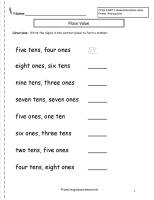Place Value Worksheet

Common Core State Standards: 2.NBT.1 Understand Place Value, Primer, Prerequesite.
Students write the number described by ones and tens. 2 digits.

###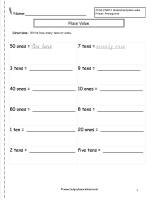Place Value Worksheet

Common Core State Standards: 2.NBT.1 Understand Place Value, Primer, Prerequesite.
Students exchange tens for ones. 2 digits.

###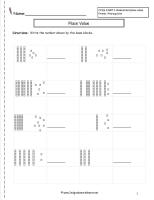Place Value Worksheet

Common Core State Standards: 2.NBT.1 Understand Place Value, Primer, Prerequesite.
Students write the number illustrated by the base ten blocks. 2 digits.

###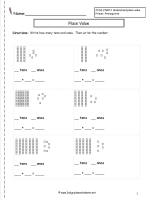Place Value Worksheet

Common Core State Standards: 2.NBT.1 Understand Place Value, Primer, Prerequesite.
Students write the number illustrated by the base ten blocks. 2 digits.

###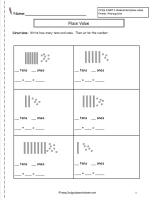Place Value Worksheet

Common Core State Standards: 2.NBT.1 Understand Place Value, Primer, Prerequesite.
Students write the number illustrated by the base ten blocks. Two digits.

###Place Value Review Worksheet

Common Core State Standards: 2.NBT.1 Understand Place Value, Primer, Prerequesite.
Two digit place value review worksheet.

###Place Value Review Worksheet

Common Core State Standards: 2.NBT.1 Understand Place Value, Primer, Prerequesite.
Two digit place value review worksheet.

###Place Value Worksheet

Common Core State Standards: 2.NBT.1 Understand Place Value
Students write number represented by the underlined digit.  3 Digits

###Place Value Worksheet

Common Core State Standards: 2.NBT.1 Understand Place Value
Students write the numbers. 3 digits.

###Place Value Worksheet

Common Core State Standards: 2.NBT.1 Understand Place Value
Students write the value of the underlined digit.  3 Digits

###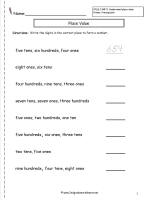Place Value Worksheet

Common Core State Standards: 2.NBT.1 Understand Place Value
Students write the number described by ones, tens, and hundreds. 3 digits.

###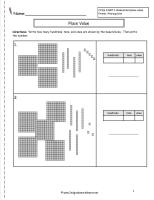Place Value Worksheet

Common Core State Standards: 2.NBT.1 Understand Place Value
Students write the number in illustrated by the base blocks.

###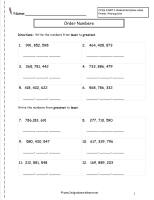Ordering Three Digit Numbers

Common Core State Standards: 2.NBT.1 Understand Place Value
Students write three 3-digit numbers in order.